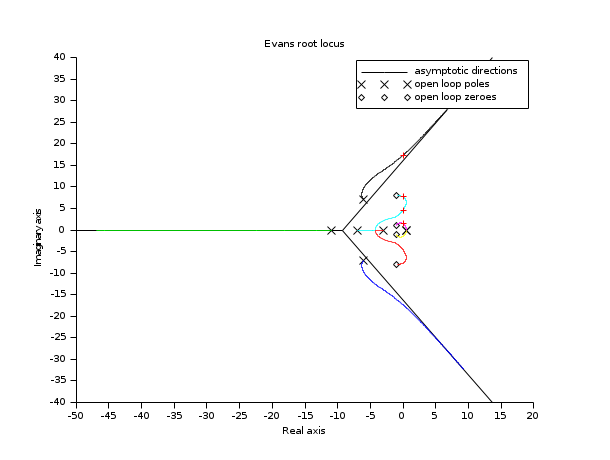Change language to:
English - Français - 日本語 - Português -

See the recommended documentation of this function

Scilab help >> CACSD > kpure

# kpure

continuous SISO system limit feedback gain

### Calling Sequence

```K=kpure(sys [,tol])
[K,R]=kpure(sys [,tol])```

### Arguments

sys

SISO linear system (syslin)

tol

a positive scalar. tolerance used to determine if a root is imaginary or not. The default value is `1e-6`

K

Real vector, the vector of gains for which at least one closed loop pole is imaginary.

R

Complex vector, the imaginary closed loop poles associated with the values of `K`.

### Description

`K=kpure(sys)` computes the gains `K` such that the system `sys` feedback by `K(i)` (`sys/.K(i)`) has poles on imaginary axis.

### Examples

```num=real(poly([-1+%i, -1-%i, -1+8*%i  -1-8*%i],'s'));
den=real(poly([0.5 0.5  -6+7*%i  -6-7*%i  -3 -7 -11],'s'));
h=num/den;

[K,Y]=kpure(h)
clf();evans(h)
plot(real(Y),imag(Y),'+r')``````num=real(poly([-1+%i*1, -1-%i*1, 2+%i*8  2-%i*8 -2.5+%i*13 -2.5-%i*13],'s'));
den=real(poly([1 1 3+%i*3 3-%i*3 -15+%i*7  -15-%i*7  -3 -7 -11],'s'));
h=num/den;

[K,Y]=kpure(h)
clf();evans(h,100000)
plot(real(Y),imag(Y),'+r')```